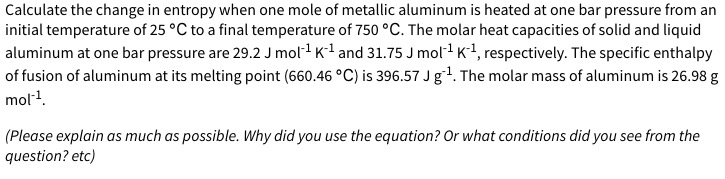# Calculate the change in entropy when one mole of metallic aluminum is heated at one bar pressure from aninitial temperature of 25 °C to a final temperature of 750 °C. The molar heat capacities of solid and liquidaluminum at one bar pressure are 29.2 J mol1 K1 and 31.75 J mol1 K1, respectively. The specific enthalpyof fusion of aluminum at its melting point (660.46 °C) is 396.57 J g1. The molar mass of aluminum is 26.98 gmol1.(Please explain as much as possible. Why did you use the equation? Or what conditions did you see from thequestion? etc)

Question
158 viewshelp_outlineImage TranscriptioncloseCalculate the change in entropy when one mole of metallic aluminum is heated at one bar pressure from an initial temperature of 25 °C to a final temperature of 750 °C. The molar heat capacities of solid and liquid aluminum at one bar pressure are 29.2 J mol1 K1 and 31.75 J mol1 K1, respectively. The specific enthalpy of fusion of aluminum at its melting point (660.46 °C) is 396.57 J g1. The molar mass of aluminum is 26.98 g mol1. (Please explain as much as possible. Why did you use the equation? Or what conditions did you see from the question? etc) fullscreen
check_circle

Step 1

Enthalpy of fusion for 1 mole of Al is 10699.45 J/mol

Initial temperature = 25 degC = 298.15K K

Final temperature = 750 degC = 1023.15K

Melting point = 660.46 deg C = 933.61K

The total entropy change is as follows,

...

### Want to see the full answer?

See Solution

#### Want to see this answer and more?

Solutions are written by subject experts who are available 24/7. Questions are typically answered within 1 hour.*

See Solution
*Response times may vary by subject and question.
Tagged in

### General Chemistry